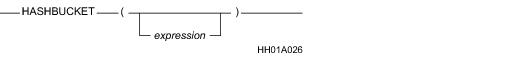# 15.00 - HASHBUCKET - Teradata Database

## Teradata Database SQL Functions, Operators, Expressions, and Predicates

Product
Release Number
15.00
Content Type
Programming Reference
Publication ID
B035-1145-015K
Language
English (United States)

## HASHBUCKET

### Purpose

Returns the hash bucket number that corresponds to a specified row hash value. If no row hash value is specified, HASHBUCKET returns the highest hash bucket number.

### Syntaxwhere:

 Syntax element … Specifies … expression an optional expression that evaluates to a valid BYTE(4) row hash value. If expression results in a UDT, Teradata Database performs implicit type conversion on the UDT, provided that the UDT has an implicit cast to a predefined byte type. To define an implicit cast for a UDT, use the CREATE CAST statement and specify the AS ASSIGNMENT clause. For more information on CREATE CAST, see SQL Data Definition Language. Implicit type conversion of UDTs for system operators and functions, including HASHBUCKET, is a Teradata extension to the ANSI SQL standard. To disable this extension, set the DisableUDTImplCastForSysFuncOp field of the DBS Control Record to TRUE. For details, see Utilities: Volume 1 (A-K). For more information on implicit type conversion, see Chapter 13: “Data Type Conversions.” For information on obtaining a row hash value that you can use for expression, see “HASHROW” on page 901.

### ANSI Compliance

This is a Teradata extension to the ANSI SQL:2011 standard.

### Result

HASHBUCKET returns an INTEGER data type.

 IF expression … THEN … does not appear in the argument list HASHBUCKET returns an INTEGER value that is the highest hash bucket number. evaluates to NULL HASHBUCKET returns NULL. evaluates to a valid BYTE(4) row hash value HASHBUCKET returns the hash bucket number corresponding to the row hash value. The range of values for hash bucket numbers depends on the system setting of the hash bucket size. If the hash bucket size is 16 bits, the hash bucket numbers can have a value from 0 to 65535. If the hash bucket size is 20 bits, the hash bucket numbers can have a value from 0 to 1048575.

### Using HASHBUCKET to Convert a BYTE Type to an INTEGER Type

When a byte data type is the source type of a conversion using CAST syntax or Teradata Conversion syntax, the target data type must also be a byte type.

To convert a BYTE(1) or BYTE(2) data type to INTEGER, you can use the HASHBUCKET function.

Consider the following table definition:

`   CREATE TABLE ByteData(b1 BYTE(1), b2 BYTE(2));`
` `

To convert column b1 to INTEGER regardless of the system setting of the hash bucket size, use the following:

`   SELECT HASHBUCKET('00'XB || b1 (BYTE(4))) / ((HASHBUCKET()+1)/65536)`
`   FROM ByteData;`

To convert column b2 to INTEGER regardless of the system setting of the hash bucket size, use the following:

`   SELECT HASHBUCKET(b2 (BYTE(4))) / ((HASHBUCKET()+1)/65536)`
`   FROM ByteData;`

### Examples

The following examples assume a table T with columns C1 and C2 and possibly other columns.

### Example

If you call HASHBUCKET without an argument, it returns the maximum hash bucket.

`   SELECT HASHBUCKET();`

### Example

If you call a HASHBUCKET function with an argument of NULL, the function returns NULL.

`   SELECT HASHBUCKET(NULL);`

### Example

Building on the previous example, you can nest a call to HASHROW within a HASHBUCKET call.

Calling HASHBUCKET (HASHROW (NULL)) returns the 0 hash bucket.

`   SELECT HASHBUCKET(HASHROW(NULL));`

### Example

The following example returns the number of rows in each hash bucket where C1 and C2 are to be the primary index of T.

`   SELECT HASHBUCKET (HASHROW (C1,C2)), COUNT (*)`
`      FROM T`
`      GROUP BY 1`
`      ORDER BY 1;`

### Example

The results of the following example lists each hash bucket that has one or more rows and its corresponding primary AMP.

`   SELECT HASHAMP (HASHBUCKET (HASHROW (C1, C2))),`
`      HASHBUCKET (HASHROW (C1,C2))`
`      FROM T`
`      GROUP BY 1,2`
`      ORDER BY 1,2 ;`